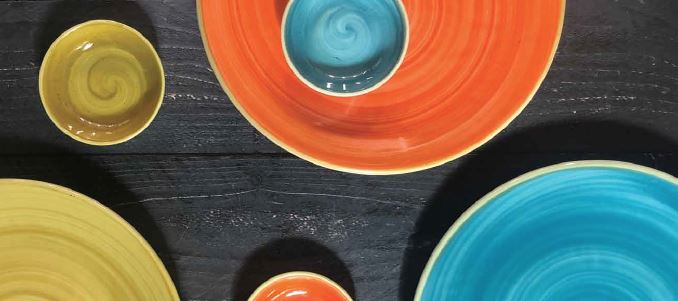Shopping Cart

Refine

View all

PriceCole & Mason£6.55 each (ex VAT)£8.90 each (ex VAT)£8.90 each (ex VAT)£7.35 each (ex VAT)£7.35 each (ex VAT)£12.99 each (ex VAT)£14.99 each (ex VAT)£10.99 each (ex VAT)£12.99 each (ex VAT)£10.20 each (ex VAT)£8.15 each (ex VAT)£8.15 each (ex VAT)£6.55 each (ex VAT)£12.99 each (ex VAT)£9.49 each (ex VAT)£12.15 each (ex VAT)£20.35 each (ex VAT)£25.50 each (ex VAT)£8.70 each (ex VAT)£10.00 each (ex VAT)£12.15 each (ex VAT)£19.69 each (ex VAT)£19.69 each (ex VAT)£8.65 each (ex VAT)£8.65 each (ex VAT)